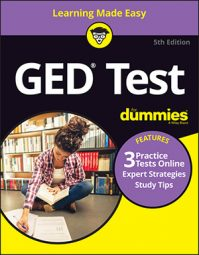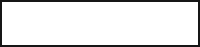##### GED Test 2022 / 2023 For Dummies with Online PracticeThe GED Math test will likely contain questions where you have to look for an algebraic pattern in a series of numbers, or use that pattern to find a missing number in the series.

Patterns are the predictable repeat of a situation. For example, if someone told you the first four numbers in a pattern were 1, 2, 3, 4 and asked you what the next number was, you’d say “5” pretty fast. This simple pattern involves adding 1 to each number to get the next one. The patterns in the following practice questions are more complicated than this one, but, if you keep your wits about you, you can figure out how to solve them.

## Practice questions

1. In the series, 4, 6, 10, 18, … , the first term that is a multiple of 11 is2. From the numbers listed, what number should go in the box?

SERIES, 4, 7, 12, 19, ____, 38, … .

A. 28 B. 26 C. 24 D. 22

1. The correct answer is 66.

This problem involves algebra, functions, and patterns. The numbers 4, 6, 10, and 18 form a pattern (also called a series). After looking carefully at the series, you see that the second term is formed by subtracting 1 from the first term and multiplying by 2. Try this on the third number:

You’ve found your pattern. Continuing the series: 4, 6, 10, 18, 34, 66, … , the first term you come to that is a multiple of 11 is 66.

You could also simply double the difference between the previous two numbers and add it to the second number to create the next one. For example, the difference between 4 and 6 is 2. Double that (2 + 2 = 4) and add it to the 6(4 + 6 = 10) to get the next number. The difference between 6 and 10 is 4. Double that (4 + 4 = 8) and add it to the 10 (8 + 10 = 18) to get the next number. Continue with this pattern until you find the number you need.

2. The correct answer is Choice (A).

This question tests your knowledge of patterns by asking you to figure out the next number in a series. By looking at the series, it looks like each number is the square of the placement of the number in the list, plus 3. That is, the first number is 12 plus 3, or 4. The second number is 22 plus 3, or 7. The third term is 32 (9) plus 3, or 12. The fifth term would be 52 (25) plus 3, which is 28.